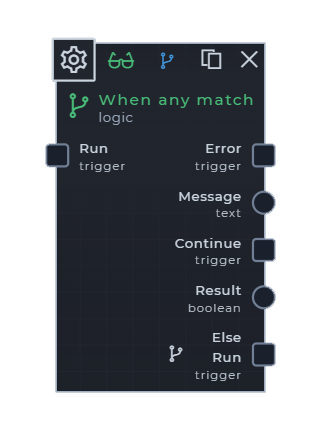# When any match

## Description

Runs the `continue` trigger when any of the input operations are true

## Input Ports

Name dataType sourceType required description
Field operations key-list manual-only false The key and operation that will happen between the key value and key predicate value

## Scenarios

• The user wants to determine if the `key` `text` value are `equals` to the `key predicate` value
• The user wants to determine if the `key` `text` value are `not equals` to the `key predicate` value.
• The user wants to determine if the `key` `text` value `contains` the `key predicate` value
• The user wants to determine if the `key` `text` value does `not contain` the `predicate` value
• The user wants to determine if the `key` `text` value is `empty`
• The user wants to determine if the `key` `text` value is `not empty`
• The user wants to determine if the `key` `number` value is `equals` to the `key predicate` value
• The user wants to determine if the `key` `number` value is `not equals` to the `key predicate` value
• The user wants to determine if the `key` `number` value is `greater than` the `key predicate` value
• The user wants to determine if the `key` `number` value is `greater than or equals` to the `key predicate` value
• The user wants to determine if the `key` `number` value is `less than` the `key predicate` value
• The user wants to determine if the `key` `number` value is `less than or equals` to the `key predicate` value
• The user wants to determine if the `key` `number` value is `empty`
• The user wants to determine if the `key` `number` value is `not empty`
• The user wants to determine if the `key` `number` value is `even`
• The user wants to determine if the `key` `number` value is `odd`
• The user wants to determine if the `key predicate` `number` value is a `multiple of` the `key` value
• The user wants to determine if the `key` `long-text` value are `equals` to the `key predicate` value
• The user wants to determine if the `key` `long-text` value are `not equals` to the `key predicate` value.
• The user wants to determine if the `key` `long-text` value `contains` the `key predicate` value
• The user wants to determine if the `key` `long-text` value does `not contain` the `key predicate` value
• The user wants to determine if the `key` `long-text` value is `empty`
• The user wants to determine if the `key` `long-text` value is `not empty`

##### With operation ports

Inputs
• Condition name: `category`
• Condition data-type: `text`
• Logic operation: `equals`
Search Tags

`Run a process with when logic` `Trigger different process` `If else` `or logic gate`